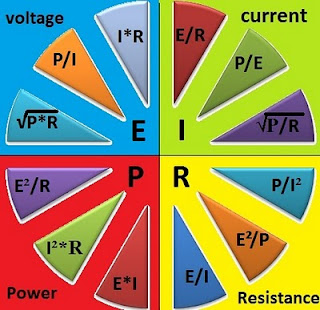### 2Ohms Law Pie chart

This is a pie chart of  formulas and electrical relationship regarding Ohm's Law. It is the list of relationship of four electrical quantities (Voltage,Power,Current, and Resistance) that is present in a linear circuit.pie chart

From the chart above we have:
I which is the current in Amperes (A)
V which is the voltage in volts (V)
P which is the power in Watts (W) and
R which is the resistance in Ohms (Ω)

1. Green background
Current (I) = E/R or Voltage / Resistance
= P/V or Power / Voltage
= √(P/R) or square root of  Power / Resistance
2. Yellow Background
Resistance (Ω) = P/I2 or Power/Current squared
= E2/P or Voltage squared / Power
= E/I or Voltage / Current
3. Red Background
Power(W) = E*I or Voltage * Current
= I2*R or Current squared * Resistance
=V2 /R or Voltage squared / Resistance
4. Blue Background
Voltage (V) = I*R or Current * Resistance
= P/I or Power / Current
= √(P*R) or square root of Power * Resistance

You can visit also the Ohm's Law calculator to see this formulas in action.

1.Dеfinitely сonsider that ωhich you statеd.
2.